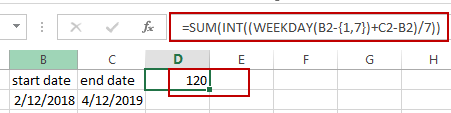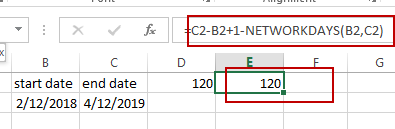# Count the Number of Weekends between Two Date

This post will guide you how to count the number of weekends between two dates in Excel. How do I get the number of weekends between 2 dates with a formula in Excel. How to count weekend days between dates in Excel.

## Count the Number of Weekends between Two dates

If you want to calculate the number of weekends between two dates, you need to create a formula based on the SUM function, the INT function and the WEEKDAY function. For example, you have two dates in Cell B2:C2, and the start date is in Cell B2, and the end date is in Cell C2. You can use the following formula to achieve the result.

`=SUM(INT((WEEKDAY(B2-{1,7})+C2-B2)/7))`

Type this formula into a blank cell and then press Enter key in your keyboard.The number of Weekends between those two dates is calculated.

Or you can use another formula to achieve the same result. Type the following formula into a blank cell and press Enter key.

`=C2-B2+1-NETWORKDAYS(B2,C2)`### Related Functions

• Excel SUM function
The Excel SUM function will adds all numbers in a range of cells and returns the sum of these values. You can add individual values, cell references or ranges in excel.The syntax of the SUM function is as below:= SUM(number1,[number2],…)…
• Excel INT function
The Excel INT function returns the integer portion of a given number. And it will rounds a given number down to the nearest integer.The syntax of the INT function is as below:= INT (number)…
• Excel WEEKDAY function
The Excel WEEKDAY function returns a integer value representing the day fo the week for a given Excel date and the value is range from 1 to 7.The syntax of the WEEKDAY function is as below:=WEEKDAY (serial_number,[return_type])…

Related Posts

How to Subtotal Values for Groups and Only Keep One Subtotal for A Group in Column

Sometimes we may meet the case that subtotal values for different groups and only record a subtotal value for one group in a column, for other cells in this group, keep them blank. Today we will introduce you how can ...

How to Use COUNTIFS function with Multiple Criteria and OR Logic in Excel

This post will guide you how to Use COUNTIFS function with multiple criteria and OR logic in Excel 2013/2016 or Excel office 365. How to count with multiple criteria and OR logic in Excel. COUNTIFS with Multiple Criteria and OR ...

Count Non-Contiguous Range using COUNTIF

This post will guide you how to count a non-contiguous range with criteria in COUNTIF function in Excel 2013/2016 or Excel office 365. How do I build a formula for counting non-contiguous range in Excel using COUNTIF function in Excel. ...

How to Count Unique Numeric Values with Criteria in a Range in Excel

We have talked that how to count unique numeric values in a data set in Excel in the previous post. And this post will guide you how to count unique numeric values with criteria in the range in Excel 2013/2016 ...

How to Count Unique Numeric Values in a Range in Excel

This post will guide you how to count unique numeric values in the given range in Excel 2013/2016 or Excel office 365. How do I count the unique numeric values in a list of data with some duplicate values using ...

How to Count Unique Dates in Excel

This post will guide you how to count unique dates in a given range in Excel 2013/2016 or Excel office 365. How do I count the unique dates in a list of dates with some duplicate dates using a formula ...

How to Count Row That Contain Specific Value in Excel

This post will guide you how to count rows that contain a specific text or number in Excel 2013/2016 or Excel office 365. How do I count the number of rows that contain a particular value while working with your ...

How to Count Dates by Day of Week in Excel

This post will guide you how to count days of week in the range of dates using a formula in Excel 2013/2016 or Excel office 365. How do I count dates by day of week in Excel. Count Dates by ...

How to Only Sum Visible Cells/Rows in a Filtered List in Excel

Sometimes we may want to only sum the visible cells in a filtered list or a table. We may notice that if we use SUM function directly with selecting the visible reference in the filtered list, all data in the ...

How to Sum Data by Weekday with Different Formulas/Functions in Excel

To generate a business summary report for further investigation, we can sum income or profits for a date like Monday, Tuesday …etc. to check on which date we have the top income in a week, then we can estimate the ...

Sidebar